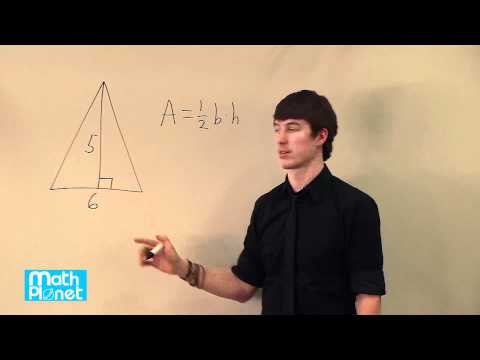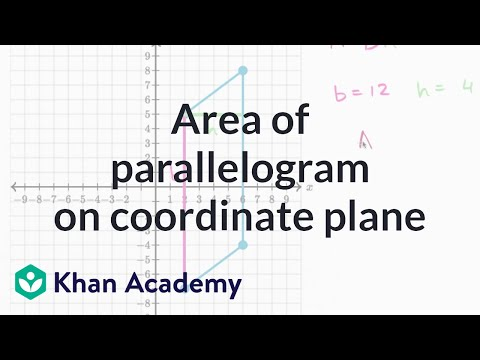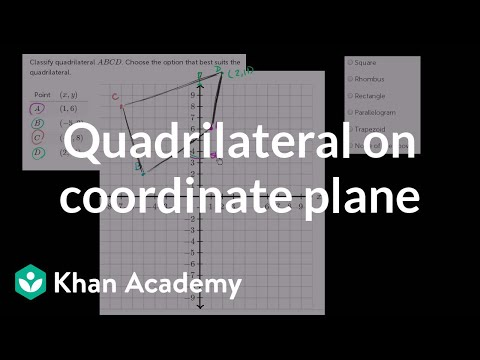# Parallelograms

##By yaymath

YouTube presents Parallelograms, an educational video resource on math.# Properties of parallelograms

##By MathPlanetVideos

Find the length of EF in the parallelogram# Parallelogram Properties

##By Textbook Tactics

YouTube presents Parallelogram Properties, an educational video resource on math.# Geometry: 6-3 Conditions for Parallelograms

##By Sir Tyler Tarver

How to prove that a given quadrilateral is a parallelogram. The great bambino.# Properties of parallelogram

##By CBSE

PROPERTIES OF PARALLELOGRAM A parallelogram is a four-sided flat shape whose opposite sides are both equal and parallel. Parallelogram has a special property of opposite sides being parallel and opposite angles being equal. A parallelogram is a quadrilateral, in which diagonals bisect each other.# Finding area of Parallelogram using determinants. - YouTube

##By TheCalculusGeek

Finding area of Parallelogram using determinants. - YouTube# Determinant and area of a parallelogram | Matrix transformations | Linear Algebra | Khan Academy

##By Khan Academy

Realizing that the determinant of a 2x2 matrix is equal to the area of the parallelogram defined by the column vectors of the matrix. All Khan Academy content is available for free at www.khanacademy.org# Proof: Diagonals of a parallelogram bisect each other | Quadrilaterals | Geometry | Khan Academy

##By Khan Academy

This video shows us how we can show that the diagonals of a parallogram bisect each other, and that any quadrilateral whose diagonals bisect each other MUST be a parallelogram.# Parallelogram, triangles etc

##By MathPlanetVideos

Find the area of the triangle# Parallelogram on the coordinate plane

##By Khan Academy

Remember our discussion of the coordinate plane? Sure you do! Let's graph the given coordinates of three of the polygon vertices and find where the 4th vertex is.# Quadrilateral problems on the coordinate plane

##By Khan Academy

Learn how to find the area of a parallelogram on the coordinate plane.# Quadrilateral angles

##By Khan Academy

Sal proves that opposite angles of a parallelogram are congruent.# Quadrilaterals: kites as a geometric shape | Quadrilaterals | Geometry | Khan Academy

##By Khan Academy

Is a kite a quadrilateral? Is it a rhombus? A parallelogram? Let's find out.# Geometry problems on the coordinate plane

##By Khan Academy

Watch Sal classify a quadrilateral as a square, rhombus, rectangle, parallelogram, or trapezoid given its four vertices.# Introduction to types of quadrilaterals | 3rd grade

##By Khan Academy

Discover attributes and features of four-sided shapes, including parallelograms, rhombuses, rectangles, and squares.# Introduction to types of quadrilaterals | 3rd grade | Khan Academy

##By Khan Academy

Discover attributes and features of four-sided shapes, including parallelograms, rhombuses, rectangles, and squares.# Quadrilateral overview | Perimeter, area, and volume | Geometry | Khan Academy

##By Khan Academy

Quad means four and lateral means line. A quadrilateral is literally a shape with four sides (lines) and four angles. Let's learn the difference between concave and convex quadrilaterals as well as trapezoids, parallelograms, and rhombi.# Quadrilaterals Overview

##By Khan Academy

"Quad" means "four" and "lateral" means "line." A quadrilateral is literally a shape with four sides (lines) and four angles. Let's learn the difference between concave and convex quadrilaterals as well as trapezoids parallelograms and rhombi.# Using 30-60-90 Triangle properties

##By Khan Academy

Khan Academy presents SAT Prep: Test 7 Section 5 Part 5, an educational video resource on math. Using 30-60-90 Triangle properties to find the diagonal of a parallelogram# Rectangle problems

##By AcademicLeadersEd

Area and perimeter problems where the sides are expressed using algebra.